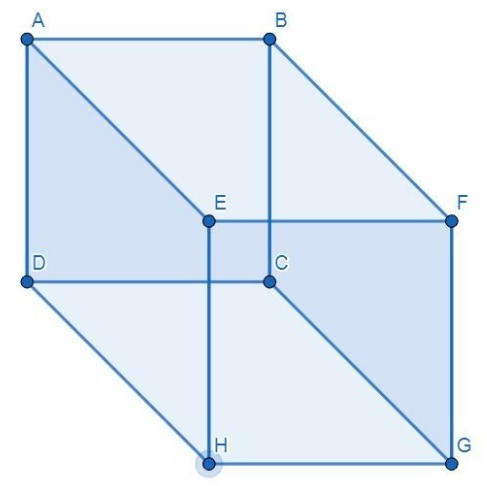QuestionAnswers

# A cuboid has length 15 feet, breadth 12 feet, and the height 10 feet. Find the total surface area of the cuboid.

Hint:First find the area of all the faces of the cuboid, that is the area of the front and back face, area of the upper and lower face, and the area of the left and right face. Then find the sum of all the faces that give the required answer.

It is given that the length, breadth and height of the cuboid is $15{\text{ feet,12 feet}}$ and $9{\text{ feet}}$ and we have to find the total surface area of the cuboid. That is,
Length$\left( l \right) = 15{\text{ feet}}$
Breadth$\left( b \right) = 12{\text{ feet}}$
Height$\left( h \right) = 10{\text{ feet}}$The total surface area of the cuboid is the sum of all the faces of the cuboid and we know that there are 6 faces in the cuboid.
Area of front and back face $\left( {{A_1}} \right) = \left( {{\text{Area of ABCD}}} \right) + \left( {{\text{Area of EFGH}}} \right)$
Area of front and back face $\left( {{A_1}} \right) = 2\left( {l \times h} \right)$
Substitute the values:
Area of front and back face $\left( {{A_1}} \right) = 2\left( {15 \times 10} \right) = 300$ square feet
Area of the upper and lower face $\left( {{A_2}} \right) = \left( {{\text{Area of ABFE}}} \right) + \left( {{\text{Area DCGH}}} \right)$
Area of the upper and lower face $\left( {{A_2}} \right) = 2\left( {l \times b} \right)$
Substitute the values:
Area of upper and lower face $\left( {{A_2}} \right) = 2\left( {15 \times 12} \right) = 360$square feet
Area of left and right face$\left( {{A_3}} \right) = \left( {{\text{Area of AEHD}}} \right) + \left( {{\text{Area of BCGF}}} \right)$
Area of left and right face $\left( {{A_3}} \right) = 2\left( {h \times b} \right)$
Substitute the values:
Area of left and right face$\left( {{A_3}} \right) = 2\left( {10 \times 12} \right) = 240$ square feet
So, the total surface area of the cuboid is given as:
The total surface area of a cuboid$= {A_1} + {A_2} + {A_3}$
Put the values:
The total surface area of a cuboid$= 300 + 360 + 240$
The total surface area of cuboid $= 900$ square feet
Therefore, the total surface area of the cuboid is $900$ square feet.

Note:We can also apply the formula of the total surface area of the cuboid to find the required result. The formula is given as:
$A = 2({\text{length}} \times {\text{breadth + breadth}} \times {\text{height + height}} \times {\text{length}})$
Substitute the values of length, breadth, and height of the cuboid into the formula:
$A = 2(15 \times 12 + 12 \times 10 + 10 \times 15)$
$A = 2\left( {180 + 120 + 150} \right)$
$A = 2\left( {450} \right)$
$A = 900$ Square feet
So, the total surface area of the cuboid is 900 square feet.